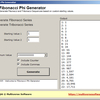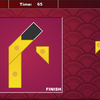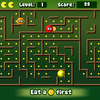# Pascal's triangle formula

Explore Pascal's Triangle visually. Pascal's Triangle Formula lets you zoom in and modify many properties of the triangle in a visual way. Pascal's Triangle is a famous and simple mathematical triangle that grows by addition. Each number is the sum of the two numbers which are directly above it. By examining the values of the triangle using modular division, many interesting patterns can result. Change the modular division value and see how each value produces a new incredible design in the triangle. Use custom color coding to generate your own interesting visual designs, or even select random colors.

## Related software (5)## Fibonacci Phi Generator

Generate custom Fibonnaci and Tribonacci sequences with this small mathematics tool. Select your own custom starting numbers. These numbers will be used to generate these special m ...## Pascal's Triangle Formula

Explore Pascal's Triangle visually. Pascal's Triangle Formula lets you zoom in and modify many properties of the triangle in a visual way. Pascal's Triangle is a famous and simple ...## Fibonacci Phi Generator

Generate custom Fibonnaci and Tribonacci sequences with this small mathematics tool. Select your own custom starting numbers. These numbers will be used to generate these special m ...## Four Piece Tangram

Can you form different shapes with only four tiles?## Math Man

Play Ghost Man with a mathematical twist!